# Tag Archives

4 Articles

## Ultimate Time Based Calculations Cheat Sheet for DAX / Power BI (including Week based calculations)

Power BI provides great time intelligence features to calculate Year-to-date (YTD), Month-to-date (MTD) and Quarter-to-date (QTD) totals. There is no such thing as Week-to-date (WTD) or Period-to-date (PTD) where period could be any arbitrary period definition (I used two-month periods in my example below). If you want those, you will have to create the calculations yourself. I was inspired by this excellent blog post and created an ultimate time-intelligence calculations Power BI file. I used Matt Massons excellent date dimension generation script to generate the date table for my example.

Basic Measures

• Total Amount:
• Total Quantity:

Day Measures

• Total Amount for Last Day (Yesterday)
• Total Amount for same Day last Year
• Variance of total Amount compared to Total Amount for Last Day
• Variance % of total Amount compared to Total Amount for Last Day
• Variance of total Amount compared to Total Amount for same Day last Year
• Variance % of total Amount compared to Total Amount same Day last Year
• Total Quantity for Last Day (Yesterday)
• Total Quantity for same Day last Year
• Variance of total Quantity compared to Total Quantity for Last Day
• Variance % of total Quantity compared to Total Quantity for Last Day
• Variance of total Quantity compared to total Quantity for same Day last Year
• Variance % of total Quantity compared to total Quantity for same Day last Year

Week Measures

• Total Amount Week To Date
• Total Amount for Last Week
• Total Amount for same Week Last Year
• Variance of total Amount Week To Date compared to Total Amount for Last Week
• Variance % of total Amount Week To Date compared to Total Amount for Last Week
• Variance of total Amount Week to Date compared to Total Amount for same Week last Year
• Variance % of total Amount Week to Date compared to Total Amount for same Week last Year
• Total Quantity Week To Date
• Total Quantity for same Week Last Year
• Total Quantity for Last Week
• Variance of total Quantity Week To Date compared to Total Quantity for Last Week
• Variance % of total Quantity Week To Date compared to Total Quantity for Last Week
• Variance of total Quantity Week to Date compared to Total Quantity for same Week last Year
• Variance % of total Quantity Week to Date compared to Total Quantity for same Week last Year

Month Measures

• Total Amount Month To Date
• Total Amount for same Month Last Year
• Total Amount for Last Month
• Variance of total Amount Month To Date compared to Total Amount for Last Month
• Variance % of total Amount Month To Date compared to Total Amount for Last Month
• Variance of total Amount Month to Date compared to Total Amount for same Month last Year
• Variance % of total Amount Month to Date compared to Total Amount for same Month last Year
• Total Quantity Month To Date
• Total Quantity for same Month Last Year
• Total Quantity for Last Month
• Variance of total Quantity Month To Date compared to Total Quantity for Last Month
• Variance % of total Quantity Month To Date compared to Total Quantity for Last Month
• Variance of total Quantity Month to Date compared to Total Quantity for same Month last Year
• Variance % of total Quantity Month to Date compared to Total Quantity for same Month last Year

Period Measures

• Total Amount Period To Date
• Total Amount for same Period Last Year
• Total Amount for Last Period
• Variance of total Amount Period To Date compared to Total Amount for Last Period
• Variance % of total Amount Period To Date compared to Total Amount for Last Period
• Variance of total Amount Period to Date compared to Total Amount for same Period last Year
• Variance % of total Amount Period to Date compared to Total Amount for same Period last Year
• Total Quantity Period To Date
• Total Quantity for same Period Last Year
• Total Quantity for Last Period
• Variance of total Quantity Period To Date compared to Total Quantity for Last Period
• Variance % of total Quantity Period To Date compared to Total Quantity for Last Period
• Variance % of total Quantity Period to Date compared to Total Quantity for same Period last Year
• Variance of total Quantity Period to Date compared to Total Quantity for same Period last Year

Quarter Measures

• Total Amount Quarter To Date
• Total Amount for same Quarter Last Year
• Total Amount for Last Quarter
• Variance of total Amount Quarter To Date compared to Total Amount for Last Quarter
• Variance % of total Amount Quarter To Date compared to Total Amount for Last Quarter
• Variance of total Amount Quarter to Date compared to Total Amount for same Quarter last Year
• Variance % of total Amount Quarter to Date compared to Total Amount for same Quarter last Year
• Total Quantity Quarter To Date
• Total Quantity for same Quarter Last Year
• Total Quantity for Last Quarter
• Variance of total Quantity Quarter To Date compared to Total Quantity for Last Quarter
• Variance % of total Quantity Quarter To Date compared to Total Quantity for Last Quarter
• Variance of total Quantity Quarter To Date compared to Total Quantity or same Quarter last Year
• Variance % of total Quantity Quarter To Date compared to Total Quantity for same Quarter last Year

Year Measures

• Total Amount Year To Date
• Total Amount for Last Year
• Variance of total Amount Year To Date compared to Total Amount for Last Year
• Variance% of total Amount Year To Date compared to Total Amount for Last Year
• Total Quantity Year To Date
• Total Quantity for Last Year
• Variance of total Quantity Year To Date compared to Total Quantity for Last Year
• Variance% of total Quantity Year To Date compared to Total Quantity for Last Year

## Power BI Pro Tip: Pareto analysis with DAX / Power Pivot

Today’s post is a guest post by Michiel Rozema (https://www.linkedin.com/in/michielrozema). Thanks Michiel!

Dutch Data Dude Jeroen approached me with the question whether it would be possible to create a Pareto chart from a Power Pivot model, using DAX. Doing a Pareto analysis using Excel is easy and numerous ways of doing it can be found online, but Jeroen wanted to use DAX formulas and could not find the solution online. I’m always in for a challenge, so here we go…

A Pareto chart (https://en.wikipedia.org/wiki/Pareto_chart) is a combo chart containing a column chart for a certain value, sorted in descending order, and a line chart with the cumulative column values, expressed as a percentage. Like this: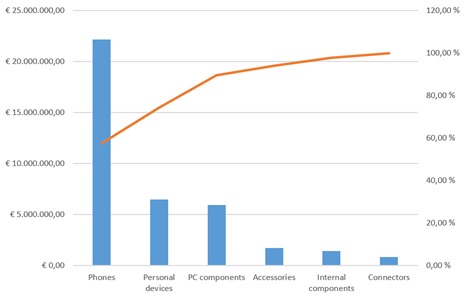The issue here is, of course, the cumulative percentage. It resembles a year-to-date total where we have months on the X-axis: for e.g. the month of May, the year-to-date total is the total for all months up to and including May. In the Pareto chart above, the percentage value for Accessories is the total of all product categories up to and including the Accessories category itself. There is no built-in DAX function for this, but as it turns out, a simple combination of a few DAX table functions does the trick; including a use of TOPN that I had not thought of before.

Let’s start with the data model. I have created a simple model with two tables, one for sales numbers and one for products: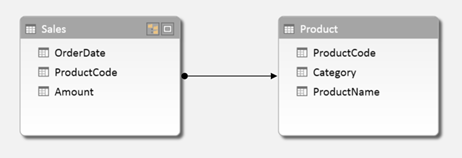We want to create a Pareto chart based on product categories, which is actually the chart shown above. For the column values in the chart, I create a basic calculated field:

For the cumulative percentage field, we need to calculate the cumulative total and divide that by the total amount for all categories. So let’s first create a calculated field for the latter one:

In this formula, ALL(Product[Category]) removes an existing filter from the Category column, therefore returning the result [TotalAmount] for all categories instead of only one.

Now it’s time to calculate the cumulative total. Let’s take the Accessories category as an example. To calculate the cumulative total for Accessories, we need to somehow determine that there are three categories placed to the left of Accessories, calculate their values, and add up the whole thing.

Remember that in the chart, the results for [TotalAmount] are shown in descending order. So we can say that for Accessories, we need to sum all categories for which [TotalAmount] is larger than the result for Accessories. If we had a Category table in our model with [TotalAmount] as a column, we could have made this calculation in a calculated column with a formula like the following:

However, we don’t have this column, [TotalAmount] cannot be a column either (we may want to add other tables to the model later on and to be able to filter the chart on customer segment, or year) and using calculated columns is not a good idea in general. So we need to take a different approach using calculated fields, and we cannot use EARLIER because we will not have a row context EARLIER can refer to.

To rephrase the cumulative total problem, we need to be able to pick some categories out of the whole list of categories based on the results of [TotalAmount]. There is a DAX function that can do this: TOPN. The obvious use of TOPN is to do calculation on for instance the top 10 customers, but in this case we will use a variable value of N in TOPN. Taking Accessories as an example again, we need to calculate the total amount for the top 4 categories. But to do that, we need to determine that Accessories is the number 4 category when it comes to [TotalAmount]. For this, we use another table function, RANKX. So we first create the calculated field below:

What does RANKX do? To quote the Power Pivot tool tip, it ‘Returns the rank of an expression in the current context in the list of values for the expression evaluated for each row in the specified table’. So, our calculation evaluates [TotalAmount] in the current context (in our example, the Accessories category), then loops through the rows of ALL(Product[Category]), which is a list of all categories (remember that ALL is a table function, and we need to use ALL because of the current context), and evaluates [TotalAmount] for each category. It then returns the rank of the result for Accessories in the list for all categories. Below is the list of results of [TotalAmount] for all categories: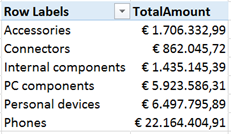When we sort the list in descending order, we can see that indeed, Accessories is the 4th category: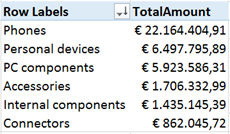With the rank, we can now calculate the cumulative total using the TOPN function:

The calculated table we use in this SUMX statement:

returns, in our example Accessories category, the four categories with the largest value of [TotalAmount]. The SUMX itself sums the [TotalAmount] values of these four categories.

Now, the only thing left to do is to calculate the Pareto percentage:

In the chart, we sort on the [TotalAmount] field used for the columns, and put [Pareto%Category] as a line chart on the secondary axis.

Creating a Pareto analysis on the Product level works exactly the same, obviously, the only difference is that we have to take care of two columns that can filter the products, [ProductCode] and [ProductName]. The calculated fields are below:

Here’s the Pareto chart with the large number of products:Just for fun, we can add categories to the X-axis and have many Pareto charts in one. I don’t really think this makes sense, but it’s nice that it works and returns the right percentages in each category. It works this way because we used the right ALL statement in our calculations.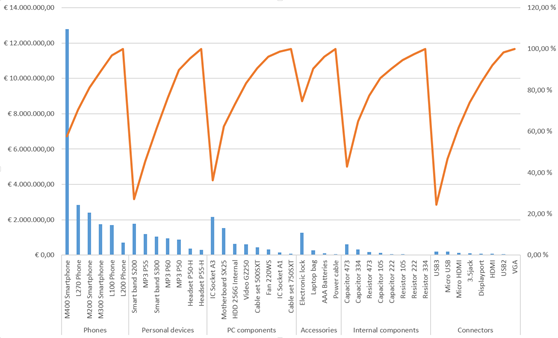So, creating a Pareto chart with mostly DAX can be done. And the combination of RANKX and TOPN turns out to be a very powerful one, which will certainly prove useful in other situations.

## Power BI Pro Tip: making date / time calculations work (Time Intelligence)

Ever so often I get asked how to do a year-over-year, quarter-over-quarter, month-over-month or year-vs-year calculation in Power BI. In most cases people would like to create a KPI to measure a certain periods performance compared to another. Power BI (specifically DAX) provides great functions for this; the Time Intelligence functions. In this scenarios PREVIOUSMONTH, PREVIOUSYEAR, SAMEPERIODLASTYEAR are used most. However, there are some frequent mistakes that result in errors when using these functions:

1) You will need to have a Date table in your model. Technically you do not need one, but you need to make sure the column you use for the time based calculations contains only unique values/dates. This is often not the case with sales happening more than once a day! Once you have the date table in the model, make sure to create a relationship between your facts date (for example sales date) and the date table.

2) The time intelligence functions should really be used as measures; not as calculated columns. This means their position in the Excel PowerPivot 2013 screen is under the horizontal line, not in the columns above.

3) Time intelligence functions work best when using totals, averages or other aggregated info.

Here are some examples. I will use ‘Date'[Date] as the reference to my date column in my date table. Also, for a best practice I split the calculation in two parts: the first part just calculates the total sales, while the other calculations refer to that base calculation.

Note that the last one uses SAMEPERIODLASTYEAR which is more flexible as it will select the same day in the previous year or the same month in the previous year depending on the selection the user makes in the tables/graphs. This is however not always what you want; so you can make it more specific by using PREVIOUSYEAR / PREVIOUSMONTH etc.

You could also use DATEADD to be even more flexible:

Notice by the way that I tend to use the short-hand notation to prevent me from having to type CALCULATE all the time (yes I am lazy :)). Here is an example of the two ways to get the sales for the previous year, the first line is the short-hand, the second is the more elaborate but not less correct option:

Hope this helps!

## Power BI Pro Tip: Confusion about TOPN() versus RANKX()

This post serves as a follow-up on my Power BI Pro Tip about using RANKX to show Top X results. I am writing this because I discovered that there is a lot of confusion about the RANKX() versus the TOPN() function.

Let me try to explain what each function does. The RANKX() function ranks individual data rows according to a certain ranking attribute. It’s result is a numerical value associated with each and every single row of the data table, as you can see below: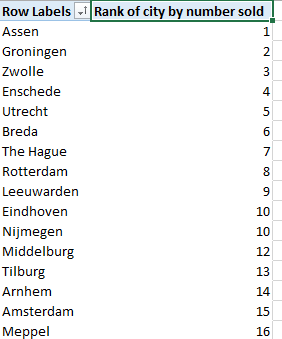Now, the TOPN() function sounds the same, right? That’s were the confusion comes from. Also, the call to the function is really similar:

RANKX( ; )

TOPN( ; ; )

However, TOPN does not return a value for each row in the data table. It returns a table that contains the top N items (N is the number you specified in the first argument) from the original data table according to the ranking attribute you specified.

In itself this is pretty useless, since you cannot display this data in any way. If you could it would be an alternative way to get a top N ranking to RANKX.

To make TOPN useful you need to wrap it in another function, such as SUMX or AVERAGEX. Let’s see an example:

MyMeasure := SUMX(TOPN(10;Sales;Sales[Sales Amount]);Sales[Sales Amount])

Now, MyMeasure equals the sum of sales amount for the best performing cities. At this point I do not know which cities it were, and maybe that is not even important to you. The total sales amount for the top 10 performers is returned.

This comes in handy when benchmarking an individual or organization against a bigger population. You could do an AVERAGEX of the TOPN result and that would be the average score for the top 10 performers. A dashboard showing how an individual measures up against the top 10 is then quickly created.